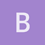Archive 04/01/2022.

Time estimates with rhosandra_oliveira

Hi,

I am using the software Network to estimate the rho ages for some mtDNA haplotypes. When I tried to calculate the rho and standard deviation (sigma) myself I found that in some cases I get different values for sigma. I am following the calculation presented in Saillard et a. 2000, just like it is done in Network. This problem seems to occur only when I have derived haplotypes that are closer to an intermediate than to the ancestral haplotype.

Consider, as an example the figure bellow. The blue haplotype is the ancestral (n=39) and the green haplotypes are derived (n=4; n=1).

From what I understand:

Rho = ( (41 ) + (12) ) / 44 = 0.13636

σ^2 = ( (4^2 * 1) + (1^2 * 2) ) / 44^2 = 0.0093

σ = 0.09642

However the value in Network is σ = 0.11589.

In a more complex example, besides the wrong estimate of sigma, I also get a different value for rho. (ancestral is in blue n=2, derived are in green n=9; 6; 1; 1; 1)

Rho= ( (92)+(63)+(13)+(13)+(1*4) ) / 20 = 2.3

σ^2 = ( ((9^2)*2) + ((6^2)*3) + ((1^2)*3) + ((1^2)*3) + ((1^2)*4) ) / 20^2

σ = 0.837Am I doing something wrong? I appreciate your help!

Sandra